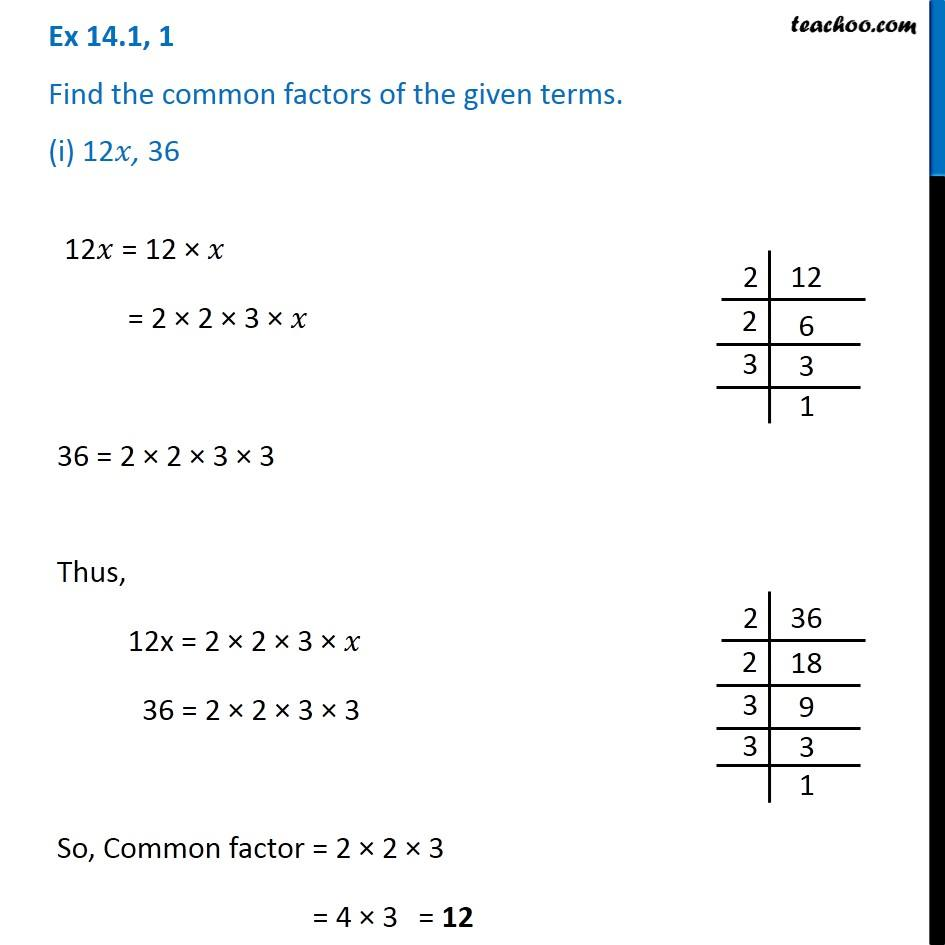Factorisation using common factors

Chapter 12 Class 8 Factorisation
Concept wiseLearn in your speed, with individual attention - Teachoo Maths 1-on-1 Class

### Transcript

Ex 12.1, 1 Find the common factors of the given terms. (i) 12𝑥, 36 12𝑥 = 12 × 𝑥 = 2 × 2 × 3 × 𝑥 36 = 2 × 2 × 3 × 3 Thus, 12x = 2 × 2 × 3 × 𝑥 36 = 2 × 2 × 3 × 3 So, Common factor = 2 × 2 × 3 = 4 × 3 = 12